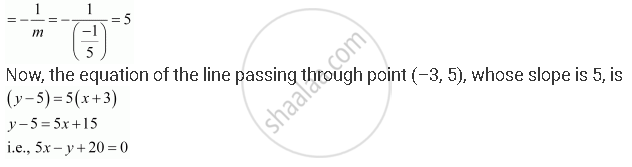CBSE (Arts) Class 11CBSE
Share

# Find the Equation of the Line Passing Through (–3, 5) and Perpendicular to the Line Through the Points (2, 5) and (–3, 6). - CBSE (Arts) Class 11 - Mathematics

ConceptVarious Forms of the Equation of a Line

#### Question

Find the equation of the line passing through (–3, 5) and perpendicular to the line through the points (2, 5) and (–3, 6).

#### Solution

The slope of the line joining the points (2, 5) and (–3, 6) is m = (6-5)/(-3-2) = 1/(-5)

We know that two non-vertical lines are perpendicular to each other if and only if their slopes are negative reciprocals of each other.

Therefore, slope of the line perpendicular to the line through the points (2, 5) and (–3, 6)Is there an error in this question or solution?

#### Video TutorialsVIEW ALL 

Solution Find the Equation of the Line Passing Through (–3, 5) and Perpendicular to the Line Through the Points (2, 5) and (–3, 6). Concept: Various Forms of the Equation of a Line.
S